Encryption & Decryption

To encrypt a message we need a method of turning a string of text into a number. We begin by ordering the alphabetic and the other key-board symbols, withfollowed by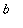,by, etc:

 > `crypt/alphabet` :=    `abcdefghijklmnopqrstuvwxyz`    ||`ABCDEFGHIJKLMNOPQRSTUVWXYZ`    ||```1234567890-=~!@#\$%^&*()_+`    ||` ,./<>?;':"[]{}|    `:

The symbols || means concatenate in Maple Version 6 and later versions. I am endebted to Professor John Cosgrave for the following function  (and its inverse) that turns a string of text into a number. (See Professor Cosgrave's home page at: www.spd.dcu.ie/johnbcos/.)

 > to_number := proc(st, string) local ll, nn, ss, ii;   ll := length(st); if ll = 0 then RETURN(0) fi; nn := 1; for ii to ll do ss := SearchText(substring(st, ii .. ii),                  `crypt/alphabet`); nn := 100*nn + ss od; nn - 10^(2*ll) end:

Next we do some examples, where the text needs to be included within backward quotes.

 > N:=to_number(`I came I saw I conquered.`);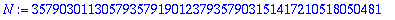The initial 35 represents the letter `I', the  79 is the blank, the 03 is the letter `c', and 81 is the period at the end.  Next we need a program to recover a string of text from the number.

 > from_number := proc(nn, integer) local ss, mm, ll, pp, ii, ans; mm := nn; ll := floor(1/2*trunc(evalf(log10(mm))))+1; ans := ``; for ii to ll do mm := mm/100; pp := 100*frac(mm); ss := substring(`crypt/alphabet`, pp..pp); ans := cat(ss, ans); mm := trunc(mm) od; ans end:

 > from_number(N);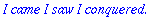It should be noted that if we use the above function on a randomly chosen number we will get gobbledegook .

 > R:=rand(10^85)();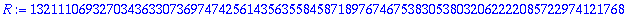> from_number(%);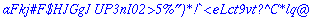Next we generate public and private keys for our friends Bob and Alice who wish to communicate privately. First for Alice and then Bob.

 > p[a]:=nextprime(rand(10^60)());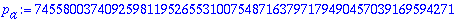> q[a]:=nextprime(rand(10^70)());> n[a]:=p[a]*q[a]; #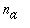is public but not the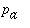and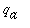.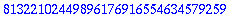> length(%);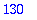> e[a]:=nextprime(12345678987654321);#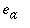is also public.> phi_na:=(p[a]-1)*(q[a]-1); # This numberis kept secret.> igcd(e[a],phi_na);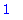> d[a]:=e[a]^(-1) mod phi_na; #is very, very private.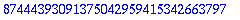> e[a]*d[a] mod phi_na;> p[b]:=nextprime(rand(10^58)());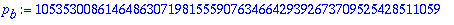> q[b]:=nextprime(rand(10^68)());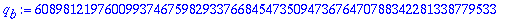> n[b]:=p[b]*q[b];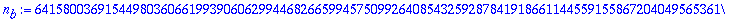> length(%);> e[b]:=nextprime(987654321234567);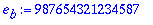> phi_nb:=(p[b]-1)*(q[b]-1);> d[b]:=e[b]^(-1) mod phi_nb;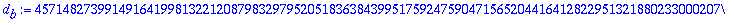Now we are ready for Bob to send a message to Alice. He first uses his secret exponentto sign his message before using Alice's public exponentto encrypt the message. First the message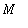.

 > M:=to_number(`Meet me at Fog City at 8:00pm. I am madly in love with you.`);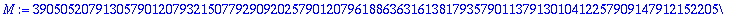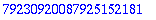> S:=M&^d[b] mod n[b];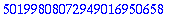> C:=S&^e[a] mod n[a];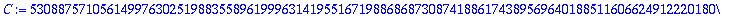Alice receives the messagefrom Bob and uses her secret exponentto decrypt. Then when she realizes that the message does not make sense and that the message is supposed to come from Bob, she uses Bob's public exponent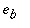to retrieve the message.

 > C&^d[a] mod n[a];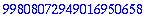> from_number(%);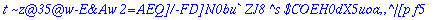> %%&^e[b] mod n[b];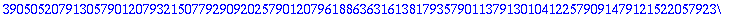> from_number(%);>

So Alice is now sure this message comes from Bob. But, will she meet him???